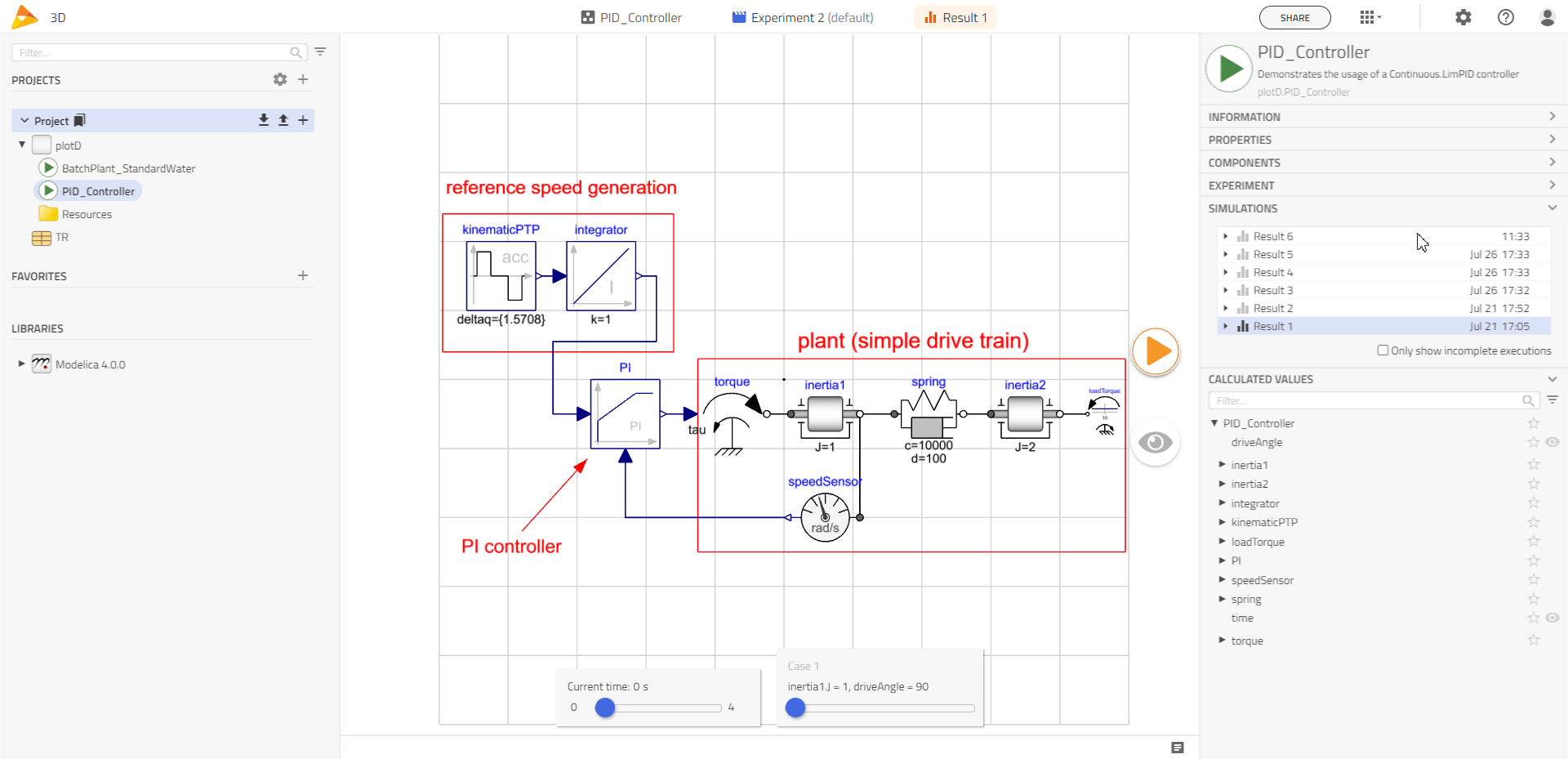# Plots🔗

Plots and visualizations are often used to display the results of simulations in a more intuitive and comprehensive manner.

To create plots after the simulation, user should typically need to follow these steps:

1. Create a plot by dragging a variable from the result browser into the model canvas.

2. A new plot is created if the variable is dragged to an empty space in the canvas.

3. If dragged onto an existing plot the variable is added to the same plot. It’s also possible to drag variables between plots.

4. The color of individual plots can be changed. Hover the cursor over the dot to the left of the name of the plot. Left-click to see the menu option. Choose a Line color.

5. Click and drag the mouse over the plot to augment the view. Repeat to further augment the view.

6. The Time Slider at the bottom of the canvas can also be used to show a line marker in each plot.

7. A plotted variable can be removed by clicking the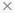button near its legend. It is possible to hide the legends (when not hovering) in a plot window by pressing the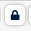button.

Create plots automation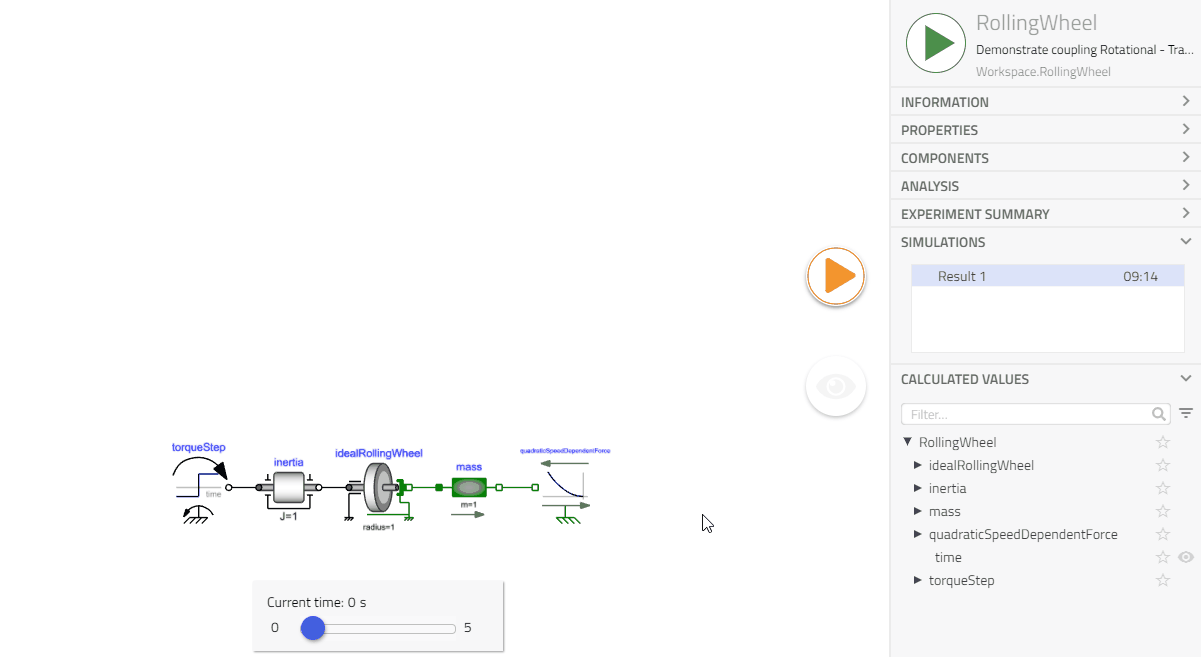### Pin Plots🔗

Pin plots help compare results between different models. Pinned plots remain on the canvas when switching between models. The legend will include the model name for the origin of the plotted variables.

1. Click on the pin icon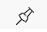to retain a plot in the canvas.

### 3D Plots🔗

To view a 3D plot, it is essential to have Multi-Execution Experiments that involve the variation of at least two variables and third variable for the other axis. The plot showcases a surface that is interpolated to cover the entire domain between the result points, providing a comprehensive view of the data. To summarize the steps:

1. Add range or choices for any two variables in Experimentation mode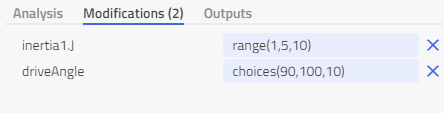1. Simulate the Model with the added range or choices

2. Create a plot by dragging a variable from the result browser into the model canvas.

3. Click on the Toggle 2D/3D icon in the plot.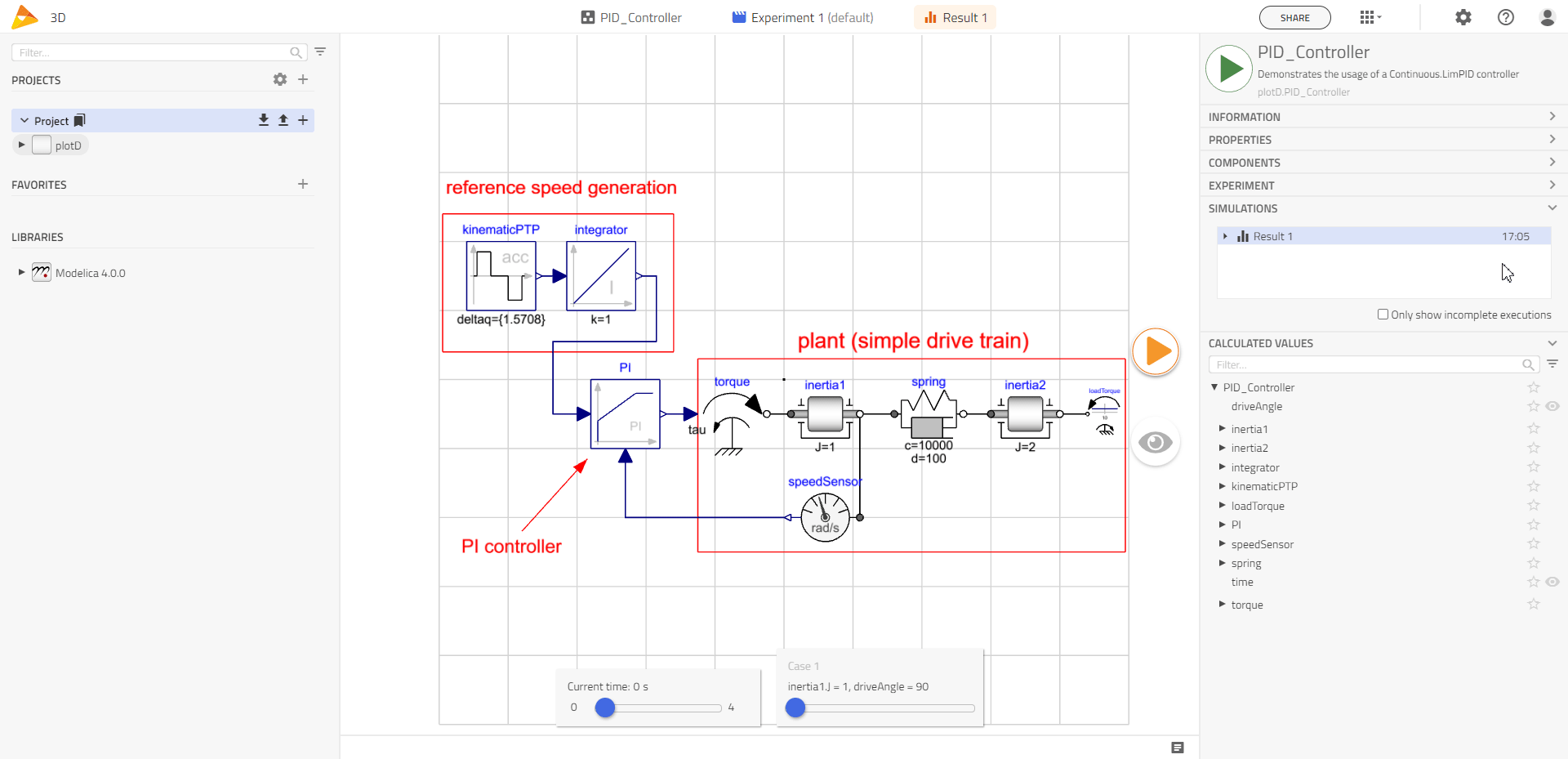1. Clicking on the Toggle 2D/3D again will switch back to 2D plot.

2. 3D plot can be downloaded by clicking on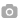button in the plot window. It will save a png image on the disk.

### Known limitations in 3D Plots🔗

1. In a 3D plot, only one result can be displayed at a time. Therefore, the plot showcases a single surface corresponding to the variation of two variables.

2. When a plot contains more than one result, the 3D button does not appear. In such cases, the plot remains in a two-dimensional format.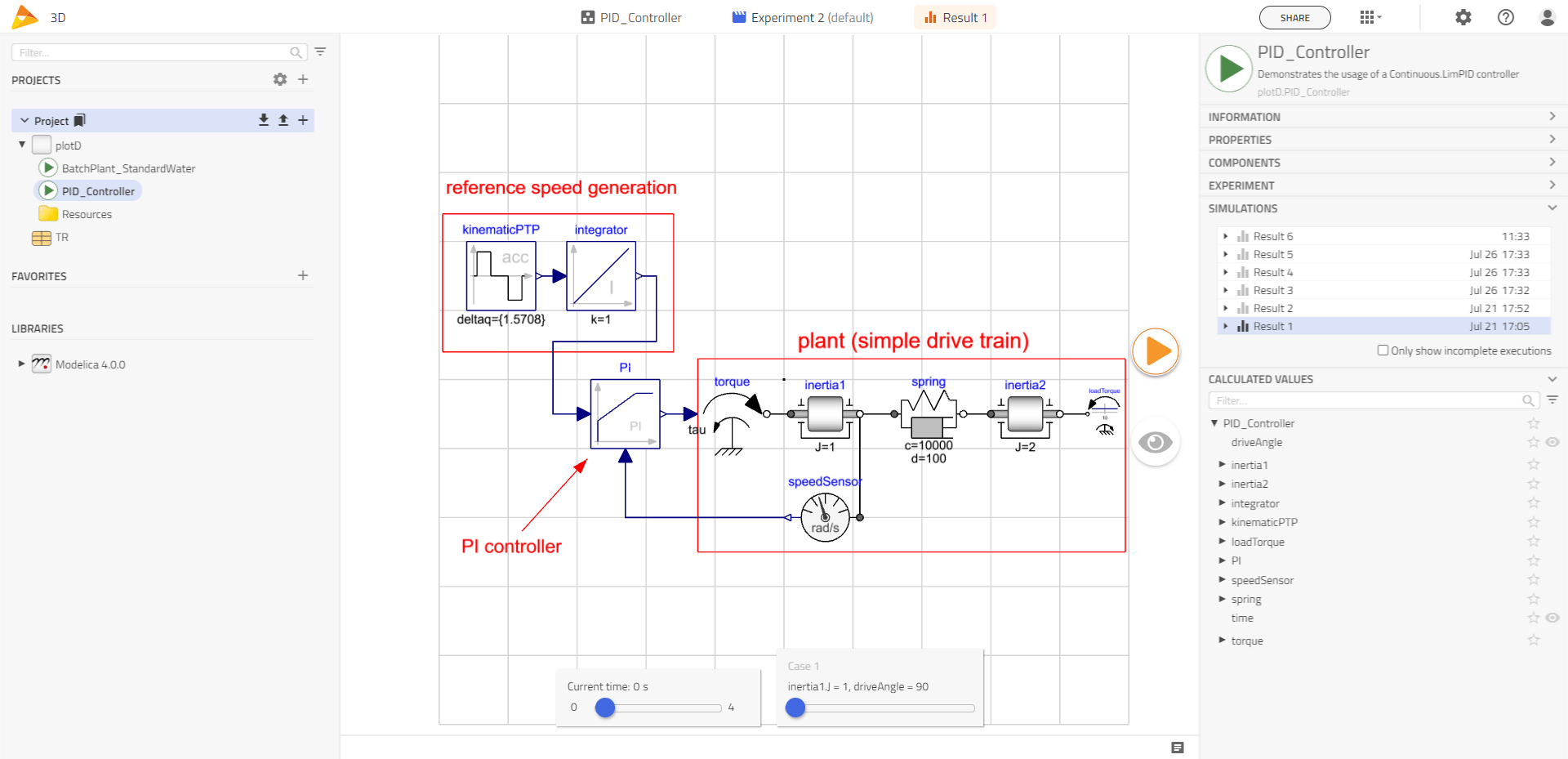1. Changing the result selection does not lead to updates in the 3D plot. In other words, when you have multiple results to choose from, the 3D plot remains unchanged. The plot retains its original presentation regardless of which result is being viewed.